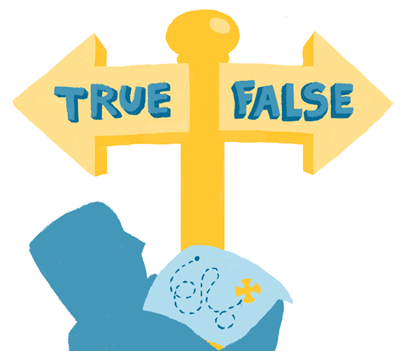The definitions of True and False in Python are defined in different versions of Python as follows.

• Python 2: None, 0, and the empty string are all counted as False, all others are True
• Python 3: None, 0, the empty string, the empty list, and the empty dictionary are all considered False, and all other values are TrueHowever, in actual use, the above definition and actual performance are not consistent. For example, the following test.

The following tests were performed in the Python 3 badlands.

 `````` 1 2 3 4 5 6 7 8 9 10 `````` ``````>>> bool(0) False >>> bool("") False >>> bool([]) False >>> bool({}) False >>> bool(None) False ``````

If the == test is used, the result is

 `````` 1 2 3 4 5 6 7 8 9 10 11 12 13 14 15 16 17 18 19 20 21 `````` ``````>>> 0 == False True >>> "" == False False >>> [] == False False >>> {} == False False >>> None == False False >>> 0 == True False >>> "" == True False >>> [] == True False >>> {} == True False >>> None == True False ``````

The main reason for the inconsistent test results is that when Python makes logical judgments, there is not only one value equal to False in Python, it also has a set of rules. The official description is.

In the context of Boolean operations, and also when expressions are used by control flow statements, the following values are interpreted as false: False, None, numeric zero of all types, and empty strings and containers (including strings, tuples, lists, dictionaries, sets and frozensets). All other values are interpreted as true. User-defined objects can customize their truth value by providing a `__bool__()` method.

The approximate logic is.

• Boolean, False for False, True for other
• integers and floating point numbers, where 0 is False and True for the rest
• String and string-like types (including bytes and unicode), False for empty strings, True for others.
• Other True sequence types (including tuples, lists, dict, sets, etc.), empty means False, non-empty means True
• None always means False

Custom types obey the following rules.

• If the `__nonzero__()` method is defined, it will be called and the return value will determine whether the object is equivalent to True or False.
• If the `__nonzero__` method is not defined but the `__len__` method is defined, the `__len__` method will be called and will be False when it returns 0, otherwise it will be True (this is the same as False when the built-in type is empty)
• If none is defined, all objects are True, only None corresponds to False

It is based on the above rules that the [PEP 8] documentation suggests something like.

 ``````1 2 3 4 5 6 7 `````` ``````For sequences, (strings, lists, tuples), use the fact that empty sequences are false. Yes: if not seq: if seq: No: if len(seq): if not len(seq): ``````